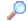Search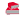Directory

Desktop Functions:

Smart Device Functions: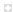Glossary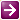Show Recent ChangesSubscribe (RSS)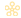Misc. Pages
ShowWindowCommand (Enums)

Summary

C# Definition:

enum ShowWindowCommands
{
/// <summary>
/// Hides the window and activates another window.
/// </summary>
Hide = 0,
/// <summary>
/// Activates and displays a window. If the window is minimized or
/// maximized, the system restores it to its original size and position.
/// An application should specify this flag when displaying the window
/// for the first time.
/// </summary>
Normal = 1,
/// <summary>
/// Activates the window and displays it as a minimized window.
/// </summary>
ShowMinimized = 2,
/// <summary>
/// Maximizes the specified window.
/// </summary>
Maximize = 3, // is this the right value?
/// <summary>
/// Activates the window and displays it as a maximized window.
/// </summary>
ShowMaximized = 3,
/// <summary>
/// Displays a window in its most recent size and position. This value
/// is similar to <see cref="Win32.ShowWindowCommand.Normal"/>, except
/// the window is not activated.
/// </summary>
ShowNoActivate = 4,
/// <summary>
/// Activates the window and displays it in its current size and position.
/// </summary>
Show = 5,
/// <summary>
/// Minimizes the specified window and activates the next top-level
/// window in the Z order.
/// </summary>
Minimize = 6,
/// <summary>
/// Displays the window as a minimized window. This value is similar to
/// <see cref="Win32.ShowWindowCommand.ShowMinimized"/>, except the
/// window is not activated.
/// </summary>
ShowMinNoActive = 7,
/// <summary>
/// Displays the window in its current size and position. This value is
/// similar to <see cref="Win32.ShowWindowCommand.Show"/>, except the
/// window is not activated.
/// </summary>
ShowNA = 8,
/// <summary>
/// Activates and displays the window. If the window is minimized or
/// maximized, the system restores it to its original size and position.
/// An application should specify this flag when restoring a minimized window.
/// </summary>
Restore = 9,
/// <summary>
/// Sets the show state based on the SW_* value specified in the
/// STARTUPINFO structure passed to the CreateProcess function by the
/// program that started the application.
/// </summary>
ShowDefault = 10,
/// <summary>
///  <b>Windows 2000/XP:</b> Minimizes a window, even if the thread
/// that owns the window is not responding. This flag should only be
/// used when minimizing windows from a different thread.
/// </summary>
ForceMinimize = 11
}

VB.NET:

Enum ShowWindowCommands As Integer
''' <summary>
''' Hides the window and activates another window.
''' </summary>
Hide = 0
''' <summary>
''' Activates and displays a window. If the window is minimized or
''' maximized, the system restores it to its original size and position.
''' An application should specify this flag when displaying the window
''' for the first time.
''' </summary>
Normal = 1
''' <summary>
''' Activates the window and displays it as a minimized window.
''' </summary>
ShowMinimized = 2
''' <summary>
''' Maximizes the specified window.
''' </summary>
Maximize = 3
' is this the right value?
''' <summary>
''' Activates the window and displays it as a maximized window.
''' </summary>
ShowMaximized = 3
''' <summary>
''' Displays a window in its most recent size and position. This value
''' is similar to <see cref="Win32.ShowWindowCommands.Normal"/>, except
''' the window is not actived.
''' </summary>
ShowNoActivate = 4
''' <summary>
''' Activates the window and displays it in its current size and position.
''' </summary>
Show = 5
''' <summary>
''' Minimizes the specified window and activates the next top-level
''' window in the Z order.
''' </summary>
Minimize = 6
''' <summary>
''' Displays the window as a minimized window. This value is similar to
''' <see cref="Win32.ShowWindowCommands.ShowMinimized"/>, except the
''' window is not activated.
''' </summary>
ShowMinNoActive = 7
''' <summary>
''' Displays the window in its current size and position. This value is
''' similar to <see cref="Win32.ShowWindowCommands.Show"/>, except the
''' window is not activated.
''' </summary>
ShowNA = 8
''' <summary>
''' Activates and displays the window. If the window is minimized or
''' maximized, the system restores it to its original size and position.
''' An application should specify this flag when restoring a minimized window.
''' </summary>
Restore = 9
''' <summary>
''' Sets the show state based on the SW_* value specified in the
''' STARTUPINFO structure passed to the CreateProcess function by the
''' program that started the application.
''' </summary>
ShowDefault = 10
''' <summary>
'''  <b>Windows 2000/XP:</b> Minimizes a window, even if the thread
''' that owns the window is not responding. This flag should only be
''' used when minimizing windows from a different thread.
''' </summary>
ForceMinimize = 11
End Enum

Notes:

None.

Documentation
ShowCmd on MSDN

Do you have...

• corrections to the existing content?
• additional languages you want to include?

Select "Edit This Page" on the right hand toolbar and edit it!Access PInvoke.net directly from VS:Terms of UseEdit This PageFind ReferencesShow Printable Version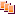Revisions Show changes